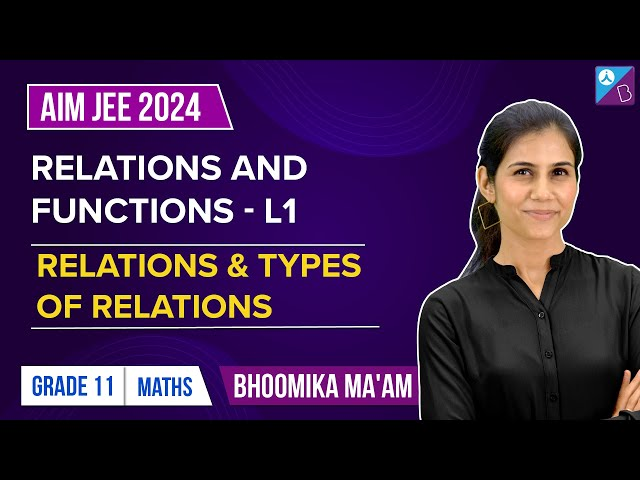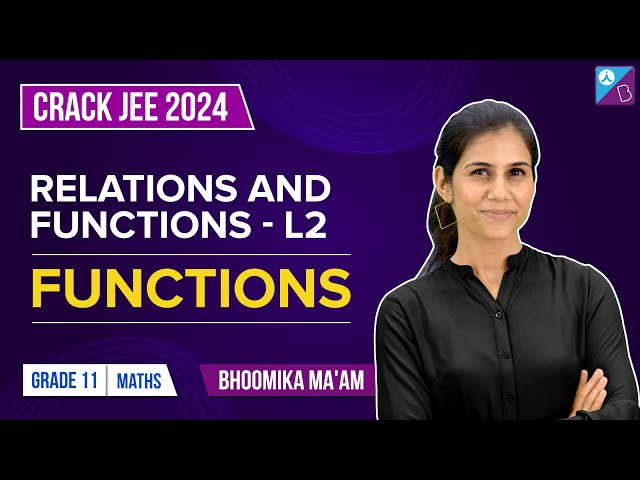# Relations and Functions Class 11

In relations and functions Class 11, you will learn the cartesian product of sets along with relations and functions. In our day-to-day life, we have known the term relations in a pattern such as the relation between brothers and sisters, husband and wife or teacher and student. In Maths, the term relation is used to relate the numbers, symbols, variables, sets, group of sets, etc. For example, A is a subset of B denotes the relation of A and B. A function is a kind of relation which is operated between two quantities to yield output.

In this article, we will provide you with the relations and functions class 11 notes, so that it would be easier for you to learn and understand the concepts.

## Relations and Functions Class 11 Chapter 2

Before we start learning about relations and functions class 11, let us go through some basics of sets. As we learned, a set is a collection of objects or elements. You have also learned about the empty set, universal set and all the other types in sets for class 11. Let us learn here, the cartesian product of sets, as this concept will be used further to explain relations and functions for class 11 solutions.

### Cartesian Product of Sets

Suppose there are two non-empty sets A and B. So, the cartesian product of A and B is the set of all ordered pairs of elements from A and B.

A × B = {(a,b) : a ∊ A, b ∊ B}

Let A = {a1, a2, a3, a4} and B = {b1, b2}

Then, The cartesian product of A and B will be;

A × B = {(a1, b1), (a2, b1), (a3, b1), (a4, b1), (a1, b2), (a2, b2), (a3, b2), (a4, b2)}

Example: Let us say, X = {a,b,c} and Y = { 1,2,3}

Therefore, X × Y = {(a, 1), (a, 2), (a, 3), (b, 1), (b, 2), (b, 3), (c, 1), (c, 2), (c, 3)}.

This set has 9 ordered pairs. We can also represent it as in a tabular form.

Note: Two ordered pair X and Y are equal, if and only if the corresponding first elements and second elements are equal.

### Relations

Definition: A relation R is the subset of the cartesian product of X x Y, where X and Y are two non-empty elements. It is derived by stating the relationship between the first element and second element of the ordered pair of X × Y. The set of all primary elements of the ordered pairs is called a domain of R and the set of all second elements of the ordered pairs is called a range of R.

For two sets X = {a, b, c} and Y = {apple, ball, cat}, the cartesian product have 9 ordered pairs, which can be written as;

X × Y = {(a, apple), (a, ball), (a, cat), (b, apple), (b, ball), (b, cat), (c, apple), (c, ball), (c, cat)}

With this we can obtain a subset of X x Y by introducing a relation R, between the elements of X and Y as;

R = {(a,b) : a is the first letter of word b, a ∊ X, b ∊ Y}

Therefore, the relation between X and Y can be represented as;

R = {(a,apple),(b,ball),(c,cat)}

Example: Let X={a,b} and Y = {c,d}. Find the number of relations from X to Y.

Solution: X × Y = {(a,c),(a,d),(b,c),(b,d)}

Number of subsets, n (X × Y) = 24 . Therefore, the number of relations from X to Y is 24.

### Functions

A relation ‘f’ is said to be a function, if every element of a non-empty set X, has only one image or range to a non-empty set Y.

Or

If ‘f’ is the function from X to Y and (x,y) ∊ f, then f(x) = y, where y is the image of x, under function f and x is the preimage of y, under ‘f’. It is denoted as;

f: X → Y.

Example: N be the set of Natural numbers and the relation R be defined as;

R = {(a,b) : b=a2, a,b ∈ N}. State whether R is a relation function or not.

Solution: From the relation R = {(a,b) : b=a2, a,b ∈ N}, we can see for every value of natural number, their is only one image. For example, if a=1 then b =1, if a=2 then b=4 and so on.

Therefore, R is a relation function here.

## Recommended Videos

### Relations and Types of Relations### Functions – Introduction, Co-domain and Range